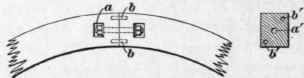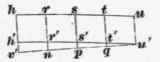Fig. 32.

The other pattern is required to trace this same curve on the concave side of the sash head. On the line w k, Fig. 33, are laid off the distances k o, or, etc. equal to c' f, etc., on the concave side of the window head in Fig. 31, and, on the perpendicular lines raised from these points, the distances k m, k n, o p, o q, r s, r t, etc. are laid off equal respectively to q b, qe, h r, h s, k t, k v, etc. of Fig. 31. The curves traced through the points n q t x and m p s w will then form the pattern for the concave side of the window frame, which must be applied in the same manner as the pattern described in Fig. 32 is applied to the outside, the line m p s w being made to conform to the straight line;n b of the concave side of the sash shown in Fig. 31.Fig. 33.

These patterns may be cut out of straw board, or thin sheet zinc, so that they may be readily bent to the curved edges of the top rail. The pattern shown in Fig. 32 is bent around the outside of the top rail, pressed closely and temporarily tacked into place, keeping l c to the joint, and the line l c h b to the straight face of the plank marked a b on the plan in Fig. 31, the convex face line c f i k being then marked on the sash head. The pattern shown in Fig. 33 is applied in the same manner to the under or concave side of the sash head, keeping the line m n to the joint and scribing the curve n t x on the outside or front edge of the sash. The surplus, or overwood, is then planed off to the convex lines so marked on the sash head, always working the plane in a direction parallel to the joint line g d', Fig. 31. When this is done and the convex face of the frame is finished, the scratch gauge is set to the thickness of the sash, and the concave or inner face of the sash is gauged from the outside. The overwood is then removed as before, moving the plane in the same direction and finishing carefully to the scribed lines. The two quadrants, each representing one-half the sash head, are now doweled together as shown at b and b' in Fig. 34, and secured with a hand-rail bolt in the center, as shown at a and a', to bring them into close contact, while a dowel in each of the two opposite corners at b' serves to keep the pieces in proper alinement. The pieces are then glued together, and the moldings are worked.

68. The arch bar may be sawed out of a single piece of plank in the same manner as each half of the window head, or it may be bent in one or more thicknesses of wood, laid out from a pattern drawn as follows: The concave side of the arch bar, Fig. 31, is divided into any number of equal parts, as h, i, j, etc., from which perpendicular lines are dropped to the plan, intersecting it at z', s; y', y; x', x, etc. At any convenient point above the plan a horizontal line v w is drawn, intersecting the perpendiculars at h' l' m', etc. Now, on a line a b, Fig. 35, the points v, h, i,j are laid off on each side of the perpendicular center line c v at a distance from each other equal to v h, h i, i j, etc., Fig. 31. From these points, the lines j x, i y, h z, etc. are drawn perpendicular to a b, and the distances j x' j x, i y' i y, h z', h z, etc. are laid off on these perpendiculars equal respectively to the distances m'x', m'x, l'y', l'y, h'z', h'z, etc., in Fig. 31. Through the points o, z', y', x', etc. and c, z, y, x, etc., curved lines are drawn giving the proper shape for the front and back edge, respectively, of a plank which is to be bent over a prepared center to form the semicircular arch bar of the sash.Fig. 34.Fig. 85.

69. The radial bars in this sash head have different curves, according to their position. No. 1 is straight, as it is in the center of the convex surface, and is parallel with the axis of the cylinder, of which the curved front of this window forms a part. No. 3 is curved to the arc of a circle whose radius is the same as the plan of the window, because it is parallel to the base of the cylinder of which the curved front forms a part. No. 2, however, being parallel to neither the axis nor the base, takes a diagonal direction around the surface of the cylinder and, therefore, is curved to the form of a portion of an ellipse.

To lay out the face mold, from which this elliptical curve may be marked on the plank for the radial bar, proceed as follows:

Divide the length of the radial bar in Fig. 31 into any number of equal parts by the points i', n', p', q' etc., and drop from each division a perpendicular line intersecting the curved plan lines at r' r", s' s", etc. Then, on a horizontal line, as v' u', Fig. 36, lay off the distances v' n, np,pq, etc., equal to i' n', n' p', p' q', etc., in Fig. 31, and layoff, on the perpendiculars erected at these points, the distances v' h, nr, ps, etc., equal to x'x", r" r', s" s', in Fig. 31, and through the points h, r, s, t, u, draw a curve which will give the outside line of the face mold required. The inside line of the face mold is then found by drawing a curve through the points h', r', s' etc., which are located so as to make the distances h h', rr', ss', etc. equal to the thickness of the sash on the lines r' r", s' s", t' t" etc. of Fig. 31.Fig. 36.

splayed work.

70. Splayed work in joinery is the term applied to cases in which some of the surfaces slope or slant away from, or towards, other surfaces, such as the sides of a pyramid. Such work is frequently encountered in the jambs, sills, or sides of windows or doors, or in the sides of any piece of work placed in an oblique direction to a horizontal or vertical plane.

71. Fig. 37 shows a box, the sides of which splay out from the bottom a b c, to the top d e f. To find the angles required in the construction of a square box, or hopper such as is shown in Fig. 37, all four of whose sides splay equally, proceed as follows: Let b c, Fig. 38, be the required splay and position of the sides of a box in relation to the horizontal plane d s, and suppose a b to be the thickness of the material from which the box is to be made; then a b c t is the end section of the material. Now, from c at right angles to d s, draw c j equal to c b; through j parallel to d s draw p h, and from b draw b h at right angles to p h; connect h c; then the angle j h c is the inside face bevel for the sides, and p h c s is the size and shape of the inside faces of the box.